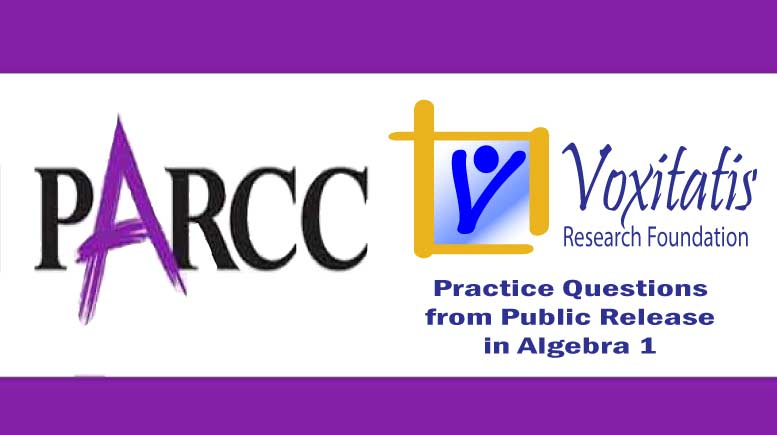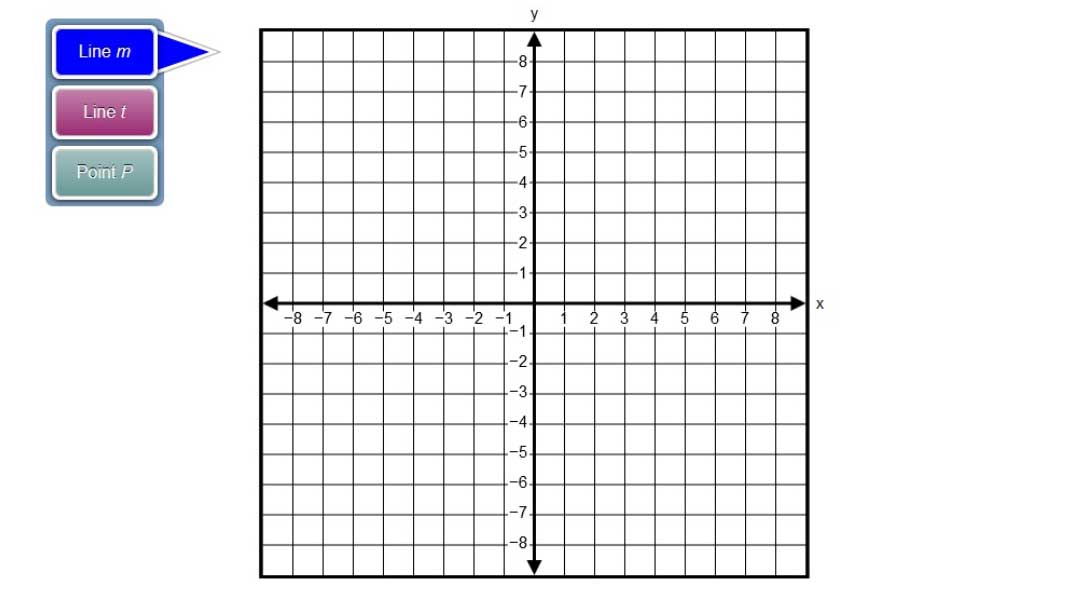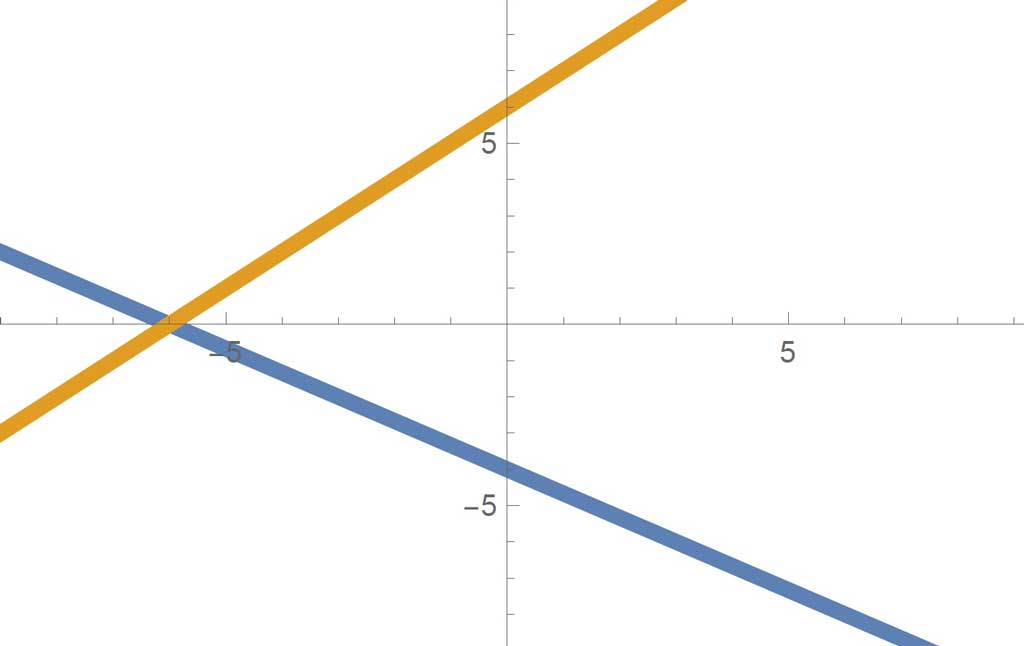Sunday, April 18, 2021# Algebra 1 PARCC question: graph y=mx+b

-#### The following technology-enabled question, explained here in hopes of helping algebra students in Maryland and Illinois prepare for the PARCC test near the end of this school year, appears on the released version of PARCC’s Spring 2015 test in algebra 1, here:

Graph lines m and t on the xy-coordinate plane shown. Then plot the point of intersection, P.

• Line m:$y = -\frac{2}{3}x - 4$
• Line t:$y = x + 6$

To graph a line, select two points on the coordinate plane. A line will be drawn through the points.

Select the place on the coordinate plane to plot the point.Answer and references

Correct answers: Line m goes through the points (–6, 0) and (0, –4). Line t goes through the points (0, 6) and (2, 8). Point P is at (–6, 0).

PARCC evidence statement(s) tested: A-REI.11-1a:

Find the solutions of where the graphs of the equations y = f(x) and y = g(x) intersect, e.g. using technology to graph the functions, make tables of values, or find successive approximations. Limit f(x) and/or g(x) to linear and quadratic functions.

The “explain” part of standard A-REI.11 is not assessed here.

The evidence statement above references Math Practice 5 and Math Practice 1 in the Common Core:  Mathematically proficient students consider the available tools when solving a mathematical problem. These tools might include pencil and paper, concrete models, a ruler, a protractor, a calculator, a spreadsheet, a computer algebra system, a statistical package, or dynamic geometry software. Proficient … high school students analyze graphs of functions and solutions generated using a graphing calculator. They detect possible errors by strategically using estimation and other mathematical knowledge. When making mathematical models, they know that technology can enable them to visualize the results of varying assumptions, explore consequences, and compare predictions with data. They are able to use technological tools to explore and deepen their understanding of concepts.

 Mathematically proficient students start by explaining to themselves the meaning of a problem and looking for entry points to its solution. They analyze givens, constraints, relationships, and goals. They make conjectures about the form and meaning of the solution and plan a solution pathway rather than simply jumping into a solution attempt. They consider analogous problems, and try special cases and simpler forms of the original problem in order to gain insight into its solution. They monitor and evaluate their progress and change course if necessary. Older students might, depending on the context of the problem, transform algebraic expressions or change the viewing window on their graphing calculator to get the information they need. Mathematically proficient students … continually ask themselves, “Does this make sense?”

The question tests students’ understanding of the eighth-grade Common Core math standard 8.F.A.3, which states that they should be able to “interpret the equation y = mx + b as defining a linear function, whose graph is a straight line,” as well as one case of 8.EE.C.8.B, which requires them to “solve systems of two linear equations in two variables algebraically, and estimate solutions by graphing the equations. Solve simple cases by inspection. For example, 3x + 2y = 5 and 3x + 2y = 6 have no solution because 3x + 2y cannot simultaneously be 5 and 6.”

Solution strategy (there are others)

Use the slope-intercept form of the equation of a line.

For Line m the slope is –⅔ and the y intercept is –4. Remember that anytime a function has the structure$y = m x + b$

you can assume that the slope of the line is m and the y intercept is b. If you put one of the points for Line m at (0, –4) and then put the second point down 2 and to the right 3, representing a slope of –⅔, the PARCC system will automatically draw the line for you.

For Line t, put the first point at the y intercept, (0, 6), as given by the equation, and then use the slope of 1 to put the second point for Line t up 1 and to the right 1, representing that slope of 1. That point could be placed at (1, 7).

The system will automatically draw Line t once the points are placed, and then you can see where the intersection occurs. Select Point P in the tool and place it on the x axis at (–6, 0).It can be determined from the graph that the two lines intersect at (–6, 0). That’s where Point P should be plotted.

It’s best to check your solution, which can be done by solving the system of equations:$y = -\frac{2}{3}x - 4$$y = x + 6$

Set the two y expressions equal to each other and solve for x:$x + 6 = -\frac{2}{3}x - 4$$\frac{5}{3}x = -10$$x = \frac{3}{5}(-10) = -6$

Then we know the point (–6, 0) is on both lines, since substituting x = –6 into the bottom equation gives us a y value of 0, as is true also for the top equation. Check.

## Resources for further study

Purple Math, developed by Elizabeth Stapel, a math teacher from the St Louis area, has a series of seven webpages that describe how to determine the solution to a system of two linear equations in two unknowns, given the equations and a way to plot the lines on a coordinate plane. The series starts here.

The Khan Academy, developed by Sal Khan, an engineer who has created a library of thousands of video lessons, has a six-part series about solving systems of linear equations, the first video in the series being entitled “Solving Linear Systems by Graphing.” It starts here.

Chapter 3, Section 3.1, of the book Algebra 2, Illinois edition by Ron Larson et al deals with solving systems of linear equations, with a nice development of how to solve (and understand) systems by graphing. The authors point out that a system of linear equations has infinitely many solutions when the two lines coincide, no solutions when the two lines are parallel, and exactly one solution in all other cases.

Complete reference: Ron Larson, Laurie Boswell, Timothy D Kanold, Lee Stiff. Algebra 2, Illinois edition. Evanston, Ill.: McDougal Littell, a division of Houghton Mifflin Company, 2008. The book is used in several algebra classes taught in Illinois high schools.

## Analysis of this question and online accessibility

The question weakly measures knowledge of the Common Core standard it purports to measure and tests students’ ability to plot lines given the slope-intercept form of the equation for the line and to estimate the point of intersection of two non-parallel, non-coincident lines by graphing. The eighth-grade standard is a prerequisite, and the use of technology is incorporated from the math practices cited. The problem is considered to have a low cognitive demand.

Once the two lines are plotted, the student is told that Point P is at the intersection of the lines. If the student knows what the word “intersection” means, the question does not assess his or her understanding of the underlying mathematics of solving a system of linear equations by graphing. This is why the question is aligned to the eighth-grade standard, not to the high school algebra standard.

The question can be tested online and should yield results that are as valid and reliable as those obtained on paper. However, the use of the tool provided by PARCC may limit students’ ability to find a solution if they are unfamiliar with the tool. It requires students to select points on a coordinate grid with a pointing device, such as a computer mouse, and then drag the two points around to obtain the desired line. If students are unfamiliar with how this tool works on the PARCC test, their ability to achieve full credit for this question could be compromised.

The Maryland and Illinois governments have passed laws giving the respective state boards of education the responsibility of adopting standards of learning for students. Good or bad, both states have adopted customized versions of the Common Core, which, in math, incorporates what are known as “math principles.” The principles, printed on the top of a 90 8-foot-wide wall chart I have in my room with the standards on it, are considered overarching. In spirit, then, no one tool is endorsed (see Math Practice 5, e.g., above), but students are expected to be creative and use whatever tool they feel is appropriate to solve a given math problem. This question forces students to use a specific technology tool to solve the problem and, as such, violates the Common Core’s prevailing math principles, which both states have duly adopted by willful action of the appropriate governing bodies. (The use of technology to estimate a solution is an artifact of PARCC and results from the consortium’s interpretation of and self-imposed restrictions on what the Common Core math practices refer to as the use of technology. This artificial limitation imposed by the test penalizes creative students, who are unable to decide for themselves which “technology” is most appropriate, as stated in the Common Core. The question, as delivered online, does not align to several key aspects of the Common Core and is therefore invalid.) No special accommodation challenges can be identified with this question, so the question is considered fair. However, if the item is delivered on paper, severe motor difficulties would present a challenge for students and would have to be accommodated. It’s possible, since human scoring would be required for paper delivery, that the item is not available on the paper version of the test. ## Challenge Given current prices and expected time of ownership, it costs about 5.5 cents per mile to operate a diesel car and about 13 cents per mile to operate a car that runs on gasoline. (Gasoline cars also require more tune-ups.) Diesel cars are also a bit more expensive than their gasoline-running counterparts. Assume a diesel car would cost you about3,500 more to purchase than a gasoline car.

If d(m) is a function that defines the cost of running a diesel car m miles after your initial purchase and g(m) is a function that defines the cost of running a gasoline car m miles after your initial (less expensive) purchase, how many miles would you drive before you break even?

## Purpose of this series of posts

Voxitatis is developing blog posts that address every algebra 1 question released to the public by the Partnership for Assessment of Readiness for College and Careers, or PARCC, in order to help students prepare to take the test this spring.

Our total release will run from February 27 through March 15, with one or two questions discussed per day. Then we’ll move to geometry at the end of March, algebra 2 during the first half of April, and eighth grade during the last half of April.Paul Katulahttps://news.schoolsdo.org
Paul Katula is the executive editor of the Voxitatis Research Foundation, which publishes this blog. For more information, see the About page.

### Md. to administer tests in math, English

0
Students in Md. will still have to take standardized tests this spring in math and English language arts, following action of the state board.